If there are 3 roads from town A to town B And 4 roads from town B to town C, in how many ways can one go from town A to town C and back to town A, through town B, without passing through the same road twice?

n =  72

### Step-by-step explanation: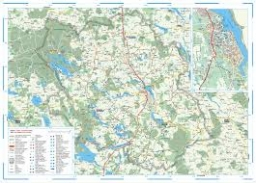Did you find an error or inaccuracy? Feel free to write us. Thank you!Tips to related online calculators
Would you like to compute count of combinations?

## Related math problems and questions: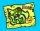There are four roads from city A to city B. There are five roads from city B to city C. How many different routes can we take from city A to city C via city B?
• Three-digit numbersUse the numbers 4,5,8,9 to write all three-digit numbers without repetition. How many such numbers are there?
• Chocolates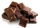How many ways can we distribute 8 different chocolates to four children?
• Three-digit numbersHow many three-digit numbers are from the numbers 0 2 4 6 8 (with/without repetition)?
• Five letters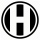How many ways can five letters be arranged?
• Four-digit numbersHow many four-digit numbers can we make from the numbers 2, 6, 3, 5, 1 and 9 if the numbers in the number cannot be repeated?
• Shelf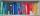How many ways are there to arrange 6 books on a shelf?
• Kids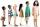How many different ways can sit 8 boys and 3 girls in line if girls want to sit on the edge?
• Three digits numberFrom the numbers 1, 2, 3, 4, 5 create three-digit numbers that digits not repeat and number is divisible by 2. How many numbers are there?
• Combinations of sweatersI have 4 sweaters two are white, 1 red and 1 green. How many ways can this done?
• DigitsHow many five-digit numbers can be written from numbers 0.3,4, 5, 7 that is divided by 10, and if digits can be repeated?
• Big numbersHow many natural numbers less than 10 to the sixth can be written in numbers: a) 9.8.7 b) 9.8.0
• Three-part novelIn how many ways can 7 books be stored on a shelf if there is one three-part novel to be placed side by side?
• Hockey Championships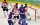At the 2021 World Hockey Championships, there are eight teams in Group A, each of which plays seven matches. There are 4 points for each team to gain points (3-2-1-0), but it is always paired with the opponent's points ( 0-1-2-3). How many points are ther
• Dinning roomHow many different combinations can we choose if there are 3 soups, 5 kinds of main dish and 2 desserts in the dining room?
• Number 4Kamila wrote all natural numbers from 1 to 400 inclusive. How many times did she write the number 4?
• Four-member teamThere are 14 girls and 11 boys in the class. How many ways can a four-member team be chosen so that there are exactly two boys in it?